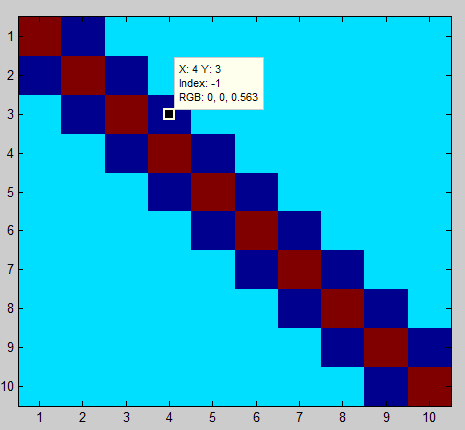# Flow control in Matlab using IF

## Introduction

This is the second lecture on what is called "control flow" in programming. We will use IF statements to control what Matlab excecutes.

## IF statemens

Help on IF statements can be found here.
```if/elseif/else

Execute statements if condition is true
Syntax
if expression
statements
elseif expression
statements
else
statements
end
Description
if expression, statements, end evaluates an expression, and
executes a group of statements when the expression is true.

elseif and else are optional, and execute statements only when
previous expressions in the if block are false.
An if block can include multiple elseif statements.
```

## Example 1

```>> a = 1;
>> if a==1
disp('Hello World')
end

Hello World
```

## Example 2

```>> b = 10;
>> if b == 10
disp('Value is exactly 10')
else
disp('Value is not 10, try again')
end

Value is exactly 10
```
```>> b = 5;
>> if b == 10
disp('Value is exactly 10')
else
disp('Value is not 10, try again')
end

Value is not 10, try again
```

## Example 3

Here is a sample script which demonstrates more complex flow control.
```X=ones(10);

if length(X)>500
disp('That is a long vector')
elseif length(X)==1
X=ones(500);
else
end
```
Video explanation:

## Example 4

This example for IF statements and For loops comes directly from the Matlab documentation on IF.
```% Preallocate a matrix
nrows = 10;
ncols = 10;
myData = ones(nrows, ncols);

% Loop through the matrix
for r = 1:nrows
for c = 1:ncols

if r == c
myData(r,c) = 2;
elseif abs(r - c) == 1
myData(r,c) = -1;
else
myData(r,c) = 0;
end

end
end

imagesc(myData)
```Video explanation:

## Example applied to Yellowstone Earthquakes

This example applies to a question from last class: How to control plotting of text.
```       if length(locations)~=0
s = sprintf('%2.1f',mean_depth);
text(long, lat, s);
end
```
Video explanation: